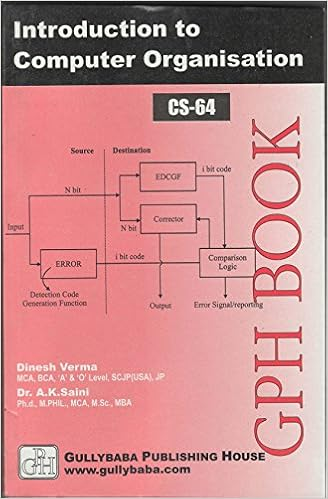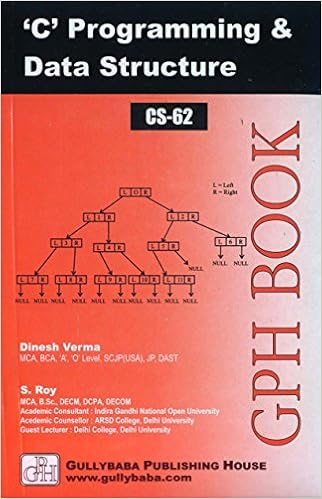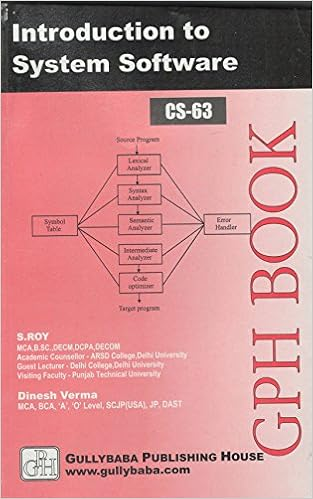IGNOU BCA MCA.comQuestion Papers of IGNOU MCA-OLD CS08

CS08: Numerical & Statical Computing
Year: 1999 TEE: june Time 3 Full Marks 75
Note: Question 1 is compulsory. Answer any three from the rest.

Q.1(a)(: Explain the purpose of COMMON and EQUIVALENCE statements in FORTRAN. Also give one example for each of these statements.

Q.1(a)(: Explain the purpose and meaning of the following FORTRAN program segment
WRITE (6, 300) K, X
300 FORMAT ( '1', 'K=', 15, T15, 'X=', F6.0): 

Q.1(a)(: For the given program segment
CHARACTER S*15; T*15
DATA S; T/'HELLO';
T = S//'THERE'
S = T(7:11)

In the above, the symbols // and : are string operations. Explain in English the meaning of each of the above four FORTRAN statements: 

Q.1(b)i: Calculate the variance for the following grouped frequency distribution

 Weight in Kilograms Number of Students 40-50 5 50-60 8 60-70home || << Previous || Next >>